# XLOOKUP Returns 0? Return Blank Instead – Excel

This tutorial will demonstrate how to return blank instead of zero when using the XLOOKUP Function. If your version of Excel does not support XLOOKUP, read how to use the VLOOKUP instead.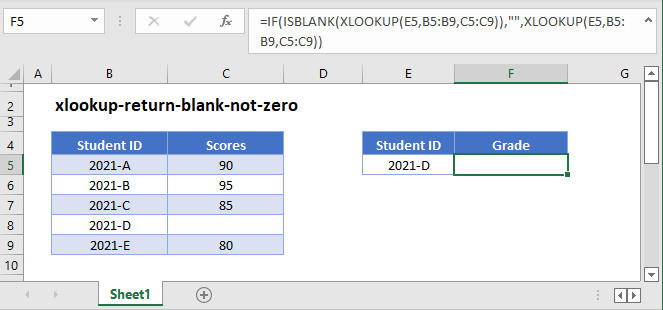In Excel, when referring to another cell with a formula, blank values are calculated as zero.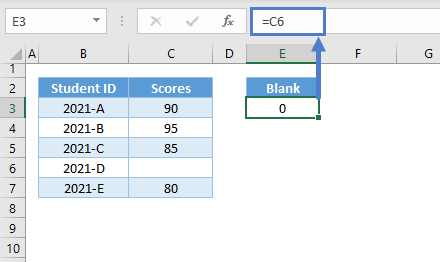This is also true when looking up values with VLOOKUP or XLOOKUP:

``=XLOOKUP("2021-D",B3:B7,C3:C7)``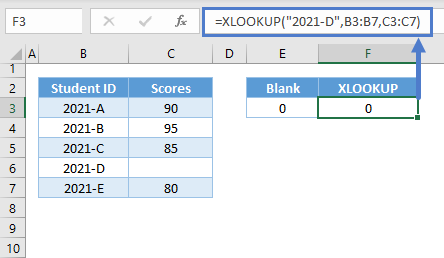This can be very problematic in some scenarios.

## XLOOKUP with IF and ISBLANK

In order to return a blank value instead of zero, we can add the IF and ISBLANK Functions to a formula:

``=IF(ISBLANK(XLOOKUP(E3,B3:B7,C3:C7)),"",XLOOKUP(E3,B3:B7,C3:C7))``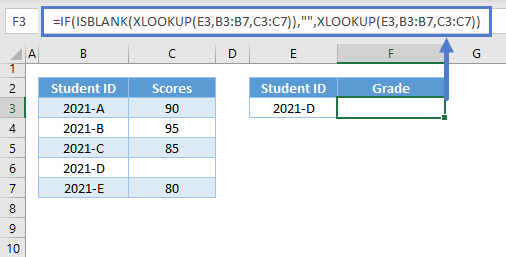Let’s walkthrough the formula:

### XLOOKUP Function

First we have our original XLOOKUP Function:

``=XLOOKUP("2021-D",B3:B7,C3:C7)``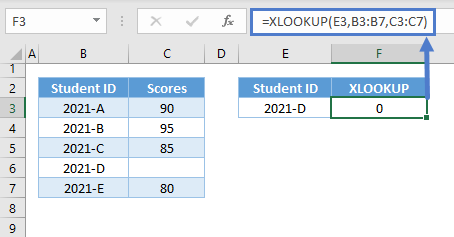### ISBLANK Function

Next, we can use ISBLANK to check if the result of the XLOOKUP Function is blank.

``=ISBLANK(XLOOKUP(E3,B3:B7,C3:C7))``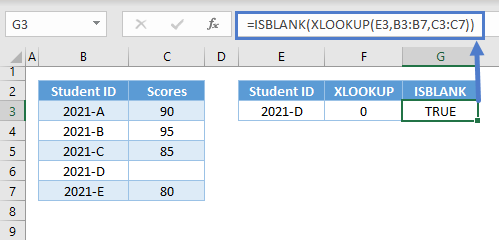Notes:

1. The ISBLANK Function returns TRUE if a value is blank. Empty string (“”) and 0 are not equivalent to a blank. A cell containing a formula is not blank, and that’s why we can’t use F3 as input for the ISBLANK.
2. Formulas can return blanks, but they are converted to zero at the final stage of the calculation.
3. Therefore, we can nest formulas in ISBLANK to check if their results are blanks rather than assigning the formulas to cells before applying ISBLANK to the cells.

### IF Function

We can then use the IF Function to test whether the combination of ISBLANK and XLOOKUP is TRUE or FALSE. We can set any process (e.g., output an empty string) if the condition is TRUE and another one (e.g., the result of the XLOOKUP if not blank) if the result is FALSE.

``=IF(G3,"",F3)``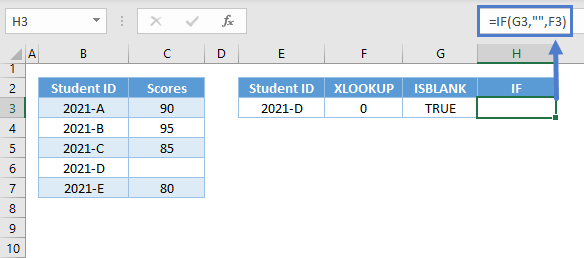Combining all of these concepts results to our original formula:

``=IF(ISBLANK(XLOOKUP(E3,B3:B7,C3:C7)),"",XLOOKUP(E3,B3:B7,C3:C7))``

## XLOOKUP with IF and Empty String (“”)

We can use the empty string as a criterion to check if the value of the XLOOKUP is blank instead of using the ISBLANK Function:

``=IF(XLOOKUP(E3,B3:B7,C3:C7)="","",XLOOKUP(E3,B3:B7,C3:C7))``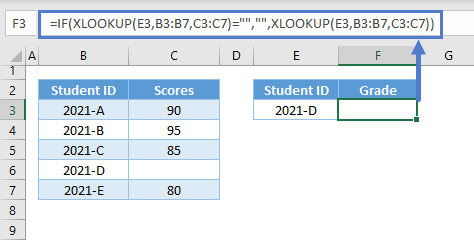Note: Blank can be equivalent to zero or empty string depending on the calculation, but empty strings and 0s are not blanks.

## XLOOKUP with IF and LEN

Another alternative to ISBLANK is the by using the LEN Function:

``=IF(LEN(XLOOKUP(E3,B3:B7,C3:C7))=0,"",XLOOKUP(E3,B3:B7,C3:C7))``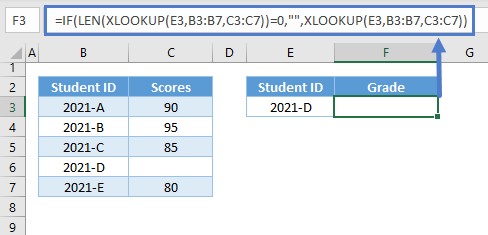Let’s dive deeper into this alternative solution:

### Easy Excel Automation### Check If Blank using LEN

We can use the LEN Function to count the number of characters of the XLOOKUP’s output:

``=LEN(XLOOKUP(E3,B3:B7,C3:C7))``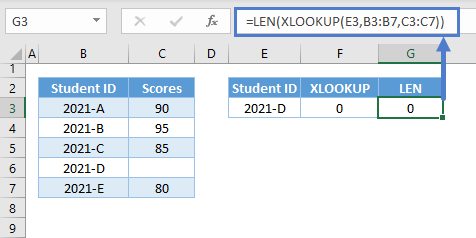If the number of characters is 0, this means that the value is blank. We can then use the IF Function to check if the LEN Function is equal to 0, and return an empty string if this is true:

``=IF(G3=0,"",F3)``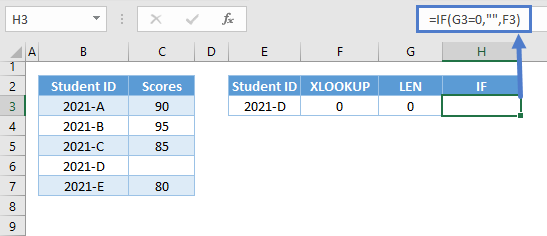Combining all of these concepts results to our original formula:

``=IF(LEN(XLOOKUP(E3,B3:B7,C3:C7))=0,"",XLOOKUP(E3,B3:B7,C3:C7))``

Take note that the formulas above are only applicable if the output is blank, which is different from a “no match” result. If XLOOKUP doesn’t find a match, it will return the #N/A Error instead, and in this case, we need to handle the #N/A Error; please see the article: “XLOOKUP – Fix #NA Error”

### Excel Practice Worksheet

Practice Excel functions and formulas with our 100% free practice worksheets!